How Cheenta works to ensure student success?
Explore the Back-Story

# Graphs in groups or Groups in graphs : TIFR GS 2018## Understand the problem

Let G be a finite group and g ∈ G an element of even order. Then we
can colour the elements of G with two colours in such a way that x and
gx have different colours for each x ∈ G.

Do you really need a hint? Try it first!

Hint 1
One needs to know the basics of Graph Theory to understand the solution.
• As noted Colouring is a fundamental topic in Graph Theory,so we need to convert the problem into a graph theory problem.
• Consider the elements of the group G as the vertices and consider edges between two elements say x and y if x=gy or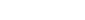. Call graph G*.
• Check that x---y---z (x is adjacent to y and y is adjacent to z) iff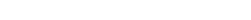or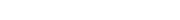.We will assume left multiplication by g ( the proof for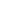is exactly the same.)
Hint 2
• Now observe that we need to answer whether this graph G* is 2-colourable.
• There is an elementary result in graph theory characterizing the 2-colourable graphs.
Theorem 1 : A graph is 2-colourable iff it is bipartite.
Theorem 2: A graph is bipartite iff it has no odd-cycle.
Hint 3 :
• Thus Theorem 1 and Theorem 2 ⇒ G* is 2 -colourable iff it has no odd cycle.
• Now what does odd cycle mean in here in terms of group.
• A path of odd length means that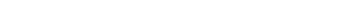in odd number of steps i.e. k is odd.
Hint 4 :
• A cycle of odd length means that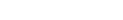.
• We are given that g is even ordered so it can only happen if k is even.
• Hence an odd cycle cannot exist and we can colour G* with 2 colours.

## Connected Program at Cheenta

College Mathematics Program the higher mathematics program caters to advanced college and university students. It is useful for I.S.I. M.Math Entrance, GRE Math Subject Test, TIFR Ph.D. Entrance, I.I.T. JAM. The program is problem driven. We work with candidates who have a deep love for mathematics. This program is also useful for adults continuing who wish to rediscover the world of mathematics.

## Understand the problem

Let G be a finite group and g ∈ G an element of even order. Then we
can colour the elements of G with two colours in such a way that x and
gx have different colours for each x ∈ G.

Do you really need a hint? Try it first!

Hint 1
One needs to know the basics of Graph Theory to understand the solution.
• As noted Colouring is a fundamental topic in Graph Theory,so we need to convert the problem into a graph theory problem.
• Consider the elements of the group G as the vertices and consider edges between two elements say x and y if x=gy or. Call graph G*.
• Check that x---y---z (x is adjacent to y and y is adjacent to z) iffor.We will assume left multiplication by g ( the proof foris exactly the same.)
Hint 2
• Now observe that we need to answer whether this graph G* is 2-colourable.
• There is an elementary result in graph theory characterizing the 2-colourable graphs.
Theorem 1 : A graph is 2-colourable iff it is bipartite.
Theorem 2: A graph is bipartite iff it has no odd-cycle.
Hint 3 :
• Thus Theorem 1 and Theorem 2 ⇒ G* is 2 -colourable iff it has no odd cycle.
• Now what does odd cycle mean in here in terms of group.
• A path of odd length means thatin odd number of steps i.e. k is odd.
Hint 4 :
• A cycle of odd length means that.
• We are given that g is even ordered so it can only happen if k is even.
• Hence an odd cycle cannot exist and we can colour G* with 2 colours.

## Connected Program at Cheenta

College Mathematics Program the higher mathematics program caters to advanced college and university students. It is useful for I.S.I. M.Math Entrance, GRE Math Subject Test, TIFR Ph.D. Entrance, I.I.T. JAM. The program is problem driven. We work with candidates who have a deep love for mathematics. This program is also useful for adults continuing who wish to rediscover the world of mathematics.

## Similar Problems

This site uses Akismet to reduce spam. Learn how your comment data is processed.

### Knowledge Partner